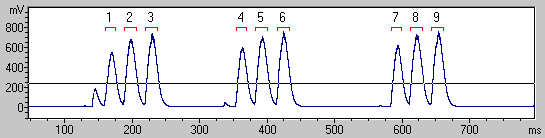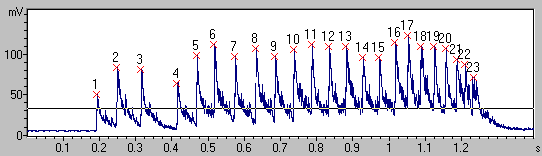### Main window : Analyze > Specials > Pulse Train Analysis Settings

This dialog box allows selecting the available pulse train analysis methods and the desired measurements.Methods

Envelope

This list box lists the available envelope calculation methods. Any quantitative analysis of waveforms requires some kind of amplitude envelope detection. For the temporal analysis of waveform patterns, it is usually not required to look at single oscillations on the original waveforms. So, it is appropriate to trace the outline of a waveform only. There are several approaches for computing the envelope or a meaningful approximation of the variation of long-term amplitude of a waveform.

Rectification + exponential decay The original waveform is first rectified (all negative samples will be inverted). The resulting envelope follows the rectified waveform as long as the amplitude is rising. If the amplitude of the rectified waveform decreases (negative slopes), the most recent peak amplitude decays exponentially at a pre-defined time constant (peak-hold mechanism). In this way, the small instantaneous amplitudes between consecutive waves will be bridged.

Rectification + exponential decay + decimation This option is identical to the above option, except that the sample rate of the resulting envelope will be reduced (decimation). The amount of decimation is selected according to the time constant of the envelope computation. The final resolution of the decimated envelope is displayed on the main Pulse Train Analysis panel. The additional decimation decreases the temporal resolution of the final measurements but accelerates the processing and displaying of the envelope, which is certainly important while processing large files.

RMS + decimation The root mean square of the waveform is computed block wise (averaging over a discrete number of samples without overlap). The inherent decimation corresponds to the ‘time constant’ parameter. In contrast to the option ‘Rectification + exponential decay’, this computational method does not include a peak-hold mechanism. Consequently, short amplitude peaks will be averaged out. Therefore, the RMS method is suited in situations where short peaks need to be rejected or are out of interest.

RMS exponential moving average+ decimation The root mean square of the waveform is computed in a moving average mode. The resulting envelope is decimated according to the selected time constant. . In contrast to the option ‘Rectification + exponential decay’, this computational method also does not include a peak-hold mechanism.

Original waveform (no manipulation) The original and unmodified soundfile is used for the peak search. Use this option for analyzing of EEG data for instance

The Envelope method can only be selected when the Pulse Train Analysis command has been launched directly from the main window waveform display. The entry points already operate on existing envelopes. The properties of these envelopes must be set before entering the Pulse Train Analysis command (e.g. by selecting appropriate Spectrogram Parameters if the Pulse Train Analysis is applied to a spectral slice of a spectrogram).

Pulse detection

There are three basic methods for detecting pulses in the amplitude envelope:

Gate Function The gate function simply compares the envelope with a pre-defined, fixed threshold. The absolute threshold level will heavily influence the number and durations of the recognized pulses. This method is recommended for analyzing signals that exhibit only minor amplitude variations.Peak Search with Hysteresis
In addition to the absolute threshold, this method is based on a relative peak detection algorithm that identifies local peaks in the envelope signal. A peak is recognized when its peak amplitude exceeds the minimum amplitude that precedes this peak by a predefined factor. In this way, it is possible to detect both soft and louder peaks that could not be detected by a simple absolute threshold comparison (Gate function). Most sound recordings contain some reverberation (short-term echoes). That reverberation can prevent the safe separation of the single sound pulses with varying amplitudes in simple gate function analysis set-ups. Therefore, this method is recommended for analyzing signals that exhibit larger amplitude variations. It is certainly useful for analyzing signals with varying amplitudes that are additionally influenced by reverberation.Valley Search with Hysteresis The valley search algorithm is similar to the above peak search, except that it detects local valleys below the threshold.

Measurements

The Measurements section allows selecting the measurements that should be taken from the pulse train.

Time Absolute time stamp of the pulse.

Time relative to group start In the group analysis mode, the relative time stamp of each pulse is referenced to the first pulse of the group.

Duration The duration is determined by the threshold settings. It is measured from the point where the signal exceeds the threshold to the point where the signal falls below the threshold level.

Interval Inter-pulse interval between pulses, measured from the start of the preceding pulse to the start of the current pulse.

Amplitude The peak amplitude of the pulse. The dB option will output result in dB units.

Energy The total energy of the pulse in Volt2*sec units.

Peak frequency / Bandwidth / Wiener Entropy : An FFT analysis is applied to the detected pulse in order to determine the peak (or dominant) frequency, bandwidth or Wiener entropy.

The Setup... button launches a dialog box that defines the spectral analysis settings.

Add filename The name of the current sound file is added to the results table. The related option to each row will add the filename to every row - otherwise it will only be added to the first (pulse) row. The option with path will include the entire pathname.

Group Analysis

The group analysis option allows assigning close pulses to single groups.

Time Absolute time stamp of the group (= time stamp of the first pulse in the group).
Pulses / Group Number of pulses in the group.
Pulses / Sec Pulse rate within the group (number of pulses per second).
Duration Duration of the group (from the start of the first pulse to the end of the last pulse).
Interval Interpulse interval between groups, measured from the start of the preceding group to the start of the current group.
Amplitude The peak amplitude of the group (the maximum peak within the group).

use section labels as groups If this option is checked, the groups will be defined by section labels (instead of the group time setting).

Overall Measurements

Pulse count The total number of pulses.
Pulse rate The total pulse rate (pulses per second) measured from the start of the first pulse to the start of the last pulse.

Group count The total number of groups.
Group rate The total group rate (groups per second) measured from the start of the first group to the start of the last group.

On time (abs in sec) The total duration of all pulses.
On time (rel. in %) The total duration of all pulses (except the last one) divided by the time interval from the start of the first pulse to the start of the last pulse.

referenced to the entire waveform If this option is activated, the On time (rel. in %), Pulse rate and Group rate will be referenced to the duration of the entire waveform (instead of the duration from the first to the last pulse).

Apply an upper amplitude limit If activated, pulses whose peak amplitude exceed a user-defined limit will be ignored.

Apply a minimum interval If activated, pulses that follow a detected pulse within the specified time interval will be ignored. Use this option to reject unwanted echoes or multipath signals.

Show pulse numbers If this option is activated, their number will identify the recognized pulses on the envelope display.

Take channel # This list box selects the sound file channel on which the Pulse Train Analysis is done.

DDE / LOG Launches the DDE Parameters / Log File dialog box. These settings are used for exporting the measurement results via the Copy buttons.

Default This button will reset all settings to their defaults.